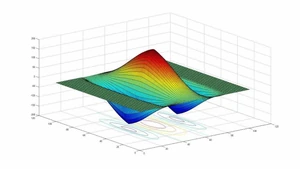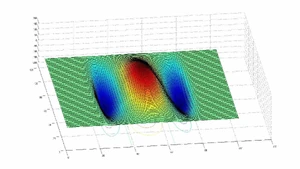## FANDOM

78 PagesA gabor filterAnother perspective

Simple Cells in Primary Visual Cortex (V1) function as Gabor filters and respond primarily to bars of particular orientation. These are formulated by the multiplication of a Gaussian function and a harmonic function. The formula that describes their function is

$G (x, y)= e^{-\pi[\frac{(x-x_0)^2}{\alpha^2}+\frac{(y-y_0)^2}{\beta^2}]}e^{-2\pi i(u_0x+v_0y)}$

Here is an example application of this filter in the proccessing of visual stimuli.